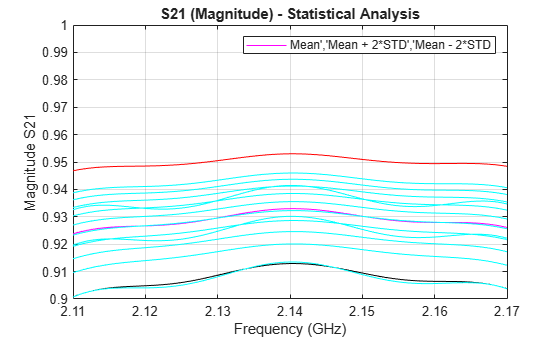# Data Analysis on S-Parameters of RF Data Files

This example shows how to perform statistical analysis on a set of S-parameter data files using magnitude, mean, and standard deviation (STD).

First, read twelve S-parameter files, where these files represent the twelve similar RF filters into the MATLAB® workspace and plot them. Next, plot and analyze the passband response of these filters to ensure they meet statistical norms.

### Read S-Parameters from Filter Data Files

Use built-in RF Toolbox™ functions for reading a set of S-Parameter data files. For each filter plot the S21 dB values. The names of the files are AWS_Filter_1.s2p through AWS_Filter_12.s2p. These files represent 12 passband filters with similar specifications.

```numfiles = 12; filename = "AWS_Filter_"+(1:numfiles)+".s2p"; % Construct filenames S = sparameters(filename(1)); % Read file #1 for initial set-up freq = S.Frequencies; % Frequency values are the same for all files numfreq = numel(freq); % Number of frequency points s21_data = zeros(numfreq,numfiles); % Preallocate for speed s21_groupdelay = zeros(numfreq,numfiles); % Preallocate for speed % Read Touchstone files for n = 1:numfiles S = sparameters(filename(n)); s21 = rfparam(S,2,1); s21_data(:,n) = s21; s21_groupdelay(:,n) = groupdelay(S,freq,2,1); end s21_db = 20*log10(abs(s21_data)); figure plot(freq/1e9,s21_db) xlabel('Frequency (GHz)') ylabel('Filter Response (dB)') title('Transmission performance of 12 filters') axis on grid on```### Filter Passband Visualization

In this section, find, store, and plot the S21 data from the AWS downlink band (2.11 - 2.17 GHz).

```idx = (freq >= 2.11e9) & (freq <= 2.17e9); s21_pass_data = s21_data(idx,:); s21_pass_db = s21_db(idx,:); freq_pass_ghz = freq(idx)/1e9; % Normalize to GHz plot(freq_pass_ghz,s21_pass_db) xlabel('Frequency (GHz)') ylabel('Filter Response (dB)') title('Passband variation of 12 filters') axis([min(freq_pass_ghz) max(freq_pass_ghz) -1 0]) grid on```### Basic Statistical Analysis of S21 Data

To determine whether the data follows a normal distribution and if there is an outlier, perform statistical analysis on the magnitude and group delay of all passband S21 data sets.

`abs_S21_pass_freq = abs(s21_pass_data);`

Calculate the mean and the STD of the magnitude of the entire passband S21 data set.

`mean_abs_S21 = mean(abs_S21_pass_freq,'all')`
```mean_abs_S21 = 0.9289 ```
`std_abs_S21 = std(abs_S21_pass_freq(:))`
```std_abs_S21 = 0.0104 ```

Calculate the mean and STD of the passband magnitude response at each frequency point. This determines if the data follows a normal distribution.

```mean_abs_S21_freq = mean(abs_S21_pass_freq,2); std_abs_S21_freq = std(abs_S21_pass_freq,0,2);```

Plot all the raw passband magnitude data as a function of frequency, as well as the upper and lower limits defined by the basic statistical analysis.

```plot(freq_pass_ghz,mean_abs_S21_freq,'m') hold on plot(freq_pass_ghz,mean_abs_S21_freq + 2*std_abs_S21_freq,'r') plot(freq_pass_ghz,mean_abs_S21_freq - 2*std_abs_S21_freq,'k') legend('Mean','Mean + 2*STD','Mean - 2*STD') plot(freq_pass_ghz,abs_S21_pass_freq,'c','HandleVisibility','off') grid on axis([min(freq_pass_ghz) max(freq_pass_ghz) 0.9 1]) ylabel('Magnitude S21') xlabel('Frequency (GHz)') title('S21 (Magnitude) - Statistical Analysis') hold off```Plot a histogram for the passband magnitude data. This determines if the upper and lower limits of the data follow a normal distribution.

```histfit(abs_S21_pass_freq(:)) grid on axis([0.8 1 0 100]) xlabel('Magnitude S21') ylabel('Distribution') title('Compare filter passband response vs. a normal distribution')```Get the groupdelay of the passband S21 data. Use inner 60% of the bandwith for statistical analysis of the groupdelay and normalize it to 10 ns.

```idx_gpd = (freq >= 2.13e9) & (freq <= 2.15e9); freq_pass_ghz_gpd = freq(idx_gpd)/1e9; % Normalize to GHz s21_groupdelay_pass_data = s21_groupdelay(idx_gpd,:)/10e-9; % Normalize to 10 ns```

Calculate the per-frequency mean and standard deviation of the normalized group delay response. All the data is collected into a single vector for alter analysis.

```mean_grpdelay_S21 = mean(s21_groupdelay_pass_data,2); std_grpdelay_S21 = std(s21_groupdelay_pass_data,0,2); all_grpdelay_data = reshape(s21_groupdelay_pass_data.',numel(s21_groupdelay_pass_data),1);```

Plot all the normalized passband groupdelay data as a function of frequency, including the upper and lower limits defined by the basic statistical analysis.

```plot(freq_pass_ghz_gpd,mean_grpdelay_S21,'m') hold on plot(freq_pass_ghz_gpd,mean_grpdelay_S21 + 2*std_grpdelay_S21,'r') plot(freq_pass_ghz_gpd,mean_grpdelay_S21 - 2*std_grpdelay_S21,'k') legend('Mean','Mean + 2*STD','Mean - 2*STD') plot(freq_pass_ghz_gpd,s21_groupdelay_pass_data,'c','HandleVisibility','off') grid on xlim([min(freq_pass_ghz_gpd) max(freq_pass_ghz_gpd)]) ylabel('Normalized group delay S21') xlabel('Frequency (GHz)') title('S21 (Normalized group delay) - Statistical Analysis') hold off```Plot a histogram for the normalized passband group delay data. This determines if the upper and lower limits of the data follow a uniform distribution.

```histogram(all_grpdelay_data,35) grid on xlabel('Group delay S21 (seconds)') ylabel('Distribution') title('Histogram of the normalized group delay')```### Analysis of Variance (ANOVA) of Data

Perform ANOVA on the magnitude of the passband S21 data.

`anova1(abs_S21_pass_freq.',freq_pass_ghz);````ylabel('Magnitude S21') xlabel('Frequency (GHz)') ax1 = gca; ax1.XTick = 0.5:10:120.5; ax1.XTickLabel = {2.11,'',2.12,'',2.13,'',2.14,'',2.15,'',2.16,'',2.17}; title('Analysis of variance (ANOVA) of passband S21 magnitude response') grid on```Perform ANOVA on the normalized groupdelay passband S21 data.

`anova1((s21_groupdelay_pass_data).',freq_pass_ghz_gpd);````ylabel('Normalized group delay S21') xlabel('Frequency (GHz)') ax2 = gca; ax2.XTick = 0.5:4:40.5; ax2.XTickLabel = {2.13,2.132,2.134,2.136,2.138,2.14,2.142,2.144,2.146,2.148,2.15}; title('Analysis of variance (ANOVA) of passband S21 groupdelay (normalized)') grid on```